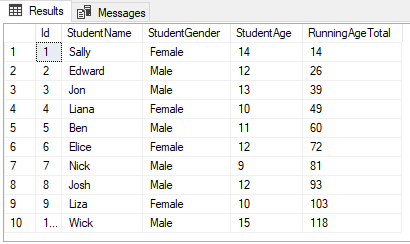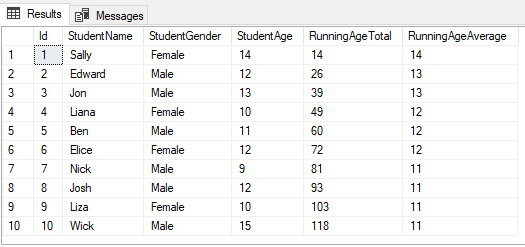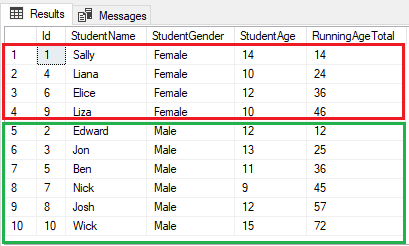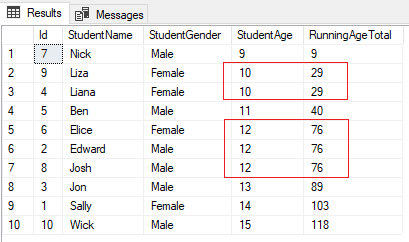# Calculating Running Total with OVER Clause and PARTITION BY Clause in SQL Server

Total: 42 Average: 4.3

You often come across scenarios where you have to calculate a running total of a quantity.

A running total refers to the sum of values in all cells of a column that precedes the next cell in that particular column.

Let’s take a look at an example to make this clearer.As you can see, the third row of the RunningAgeTotal column contains the sum of all the values in the 1st to the 3rd rows of the StudentAge column, i.e. 14 + 12 + 13 = 39.

Similarly, the value of the 4th row of the RunningAgeTotal column is 49, which is the sum of the values in the 1st to the 4th rows of the StudentAge column.

In the SQL Server, the OVER clause can be used to calculate running totals.

Let’s explore how to use this with the help of a below example.

### Simple Example of Calculating Running Total

Let’s create some dummy data before actually writing a query that calculates a running total.

First, execute the following script:

This script creates the Students table within the School database. There are four columns in the table: Id, StudentName, StudentGender, and Student. The INSERT statement adds 10 dummy records to the database.

To calculate the running total, we have to use an OVER clause and adds the column which we want to calculate the running total for. The following script calculates the running total of the values in the StudentAge column and adds the result to the RunningAgeTotal column.

In the script above, the SELECT statement retrieves the StudentName, StudentGender, and StudentAge columns along with the running total column, i.e. RunningAgeTotal. The SUM Aggregate function adds the values to the StudentAge column and the OVER clause determines that the addition should be performed in the form of running total ordered by the Id column. The output of the above script is as follows:### Calculate Running Average

You can modify the script in the last section to calculate a running average age of all the students in the Students table. To do this, execute the following script:

AS you can see, we use the AVG aggregate function to calculate the average age of all the students in the StudentAge column. The output of the above script looks like this:Take a look at the third row of the RunningAgeAverage column. It contains the average of values of the 1st to 3rd rows in the StudentAge column, i.e (14 + 12 + 13)/3 = 13.

### Partitioning Running Total by Column Values

You can also calculate a running total by partitioning data by the values in a particular column. For instance, you can calculate a running total of the students’ age, partitioned by gender. To do this, you have to use a PARTITION BY statement along with the OVER clause.

Take a look at the following example:

The only difference between calculating the running total for all records and calculating the running total by gender is the use of the PARTITION BY StudentGender clause within the parenthesis after the OVER clause. The above script calculates the running total for the values in the StudentAge column, partitioned by the values in the StudentGender column. The output looks like this.Now, take a look at the first four values in the RunningAgeTotal column (Highlighted by the red rectangle). These values are the running total of the female students. Similarly, the last 6 rows (highlighted by the green rectangle) contain a running total of the age for the male students in the Students table.

### Problems with OVER when a Column has a Duplicate Column

A problem arises if a column with duplicate values is used with an OVER clause in order to calculate a  running total. Take a look at the StudentAge column. Elice, Edward, and Josh all have the same age, i.e. 12. Similarly, Liana and Liza also have the same values in the StudentAge column, i.e. 10.

If you try to calculate a running total by specifying the StudentAge column within the parenthesis after the OVER clause, you will see some weird results. Let’s run this query:

The output of the above query is as follows:In the second row of the RunningAgeTotal column, the value is 29. However, it should be 19 because the 1st and 2nd rows of the StudentAge column contain 9 and 10 respectively. In this case, since both 2nd and 3rd rows of the StudentAge column contain a duplicated value, i.e. 10, the value for the 2nd row of the RunningAgeTotal column is calculated by adding 9, 10, and 10. Similarly, for the 3rd row of the RunningAgeTotal column, the value from the second row that is 29 is used.

Likewise, if you look at the 5th row of the RunningAgeTotal column, the value is 76. It should actually be 40 + 12 = 52. However, since the 5th, 6th, and 7th rows of the StudentAge column have duplicate values,  i.e. 12, the running total is calculated by adding 40 + 12 + 12 + 12 = 76. This running total has been used for the rows 6th and 7th of the RunningAgeTotal column because the rows 6th and 7th of the StudentAge column contain the duplicate values as the row 5.

To avoid this situation, you need to stop using columns with duplicate values along with the OVER clause. The Primary Key column is always a good choice to be used with the OVER clause since it only contains unique values.